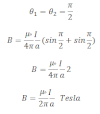# Magnetic Field Due To Straight Current Carrying Conductor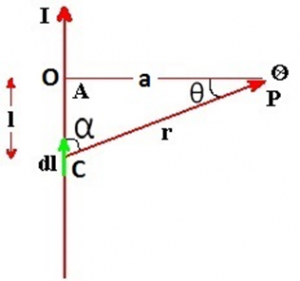Consider an infinitely long conductor AB through which current I flows. Let P be any point at a distance a from the centre of conductor. Consider dl be the small current carrying element at point c at a distance r from point p. α be the angle between r and dl. l be the distance between centre of the coil and elementary length dl.
From biot-savart law, magnetic field due to current carrying element dl at point P is: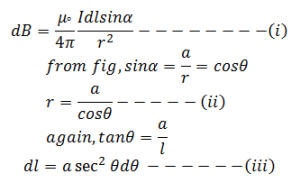From above three equations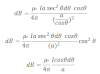Total magnetic field due to straight current carrying conductor is: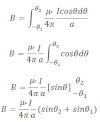This is the final expression for total magnetic field due to straight current carrying conductor.

If the conductor having infinite length then,